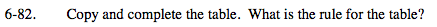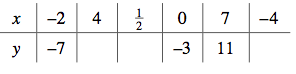### Home > MC2 > Chapter 6 > Lesson 6.2.1 > Problem6-82

6-82.Look for any x-values of 0 to find some sort of constant. In this case, an x-value of 0 equates to a y-value of -3, so we can include the -3 value in the rule.

Since subtracting 3 does not give the correct y-value for the rest of the table, there are more parts to this rule.

We can now try working backwards to find the other missing parts. If the y-value was obtained by subtracting 3 among other things, we can add 3 to the y-value and see what other changes took place as well.

Using the x-value −2 and y-value −7:
Adding 3 to −7 = −4
−4 is two times −2, the x -value.

By adding 2x to the rule, we get y = 2x − 3.

Test to see if this rule is correct by substituting in 7 for the x-value and see if you get 11 as the y-value. If it is, use the rule to fill in the rest of the table. One value has been given to you (when x = 4, y = 5).